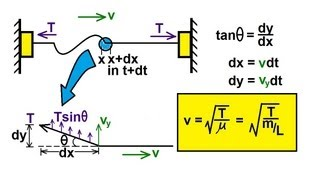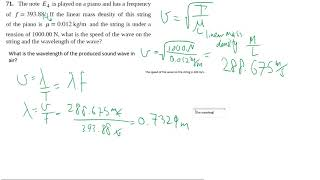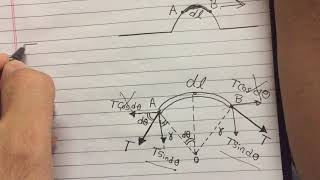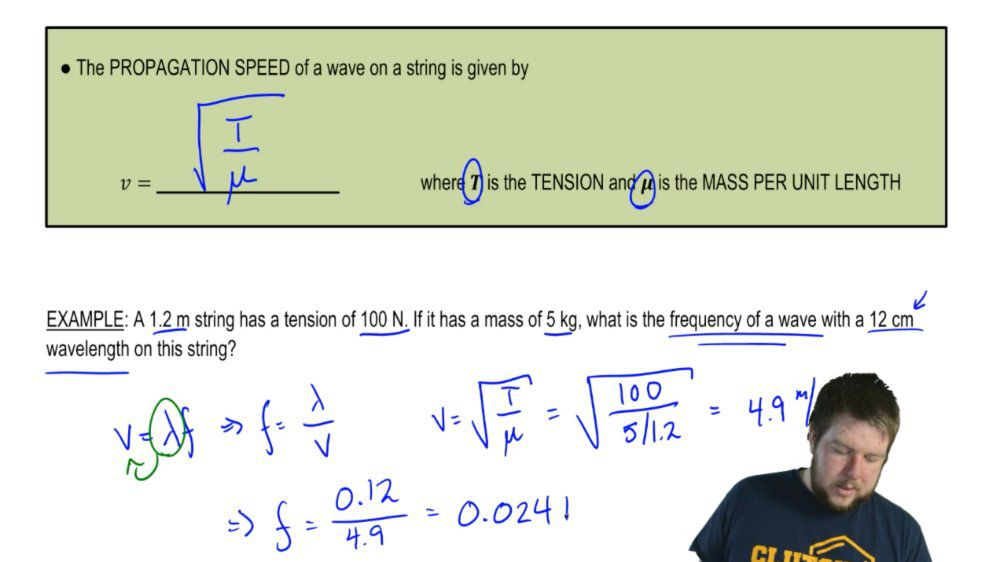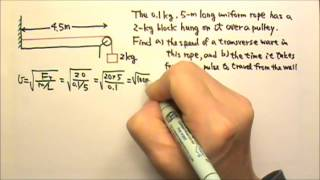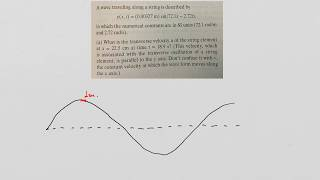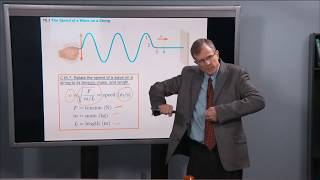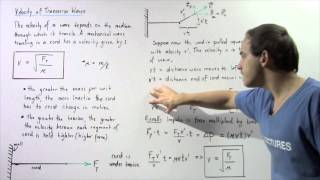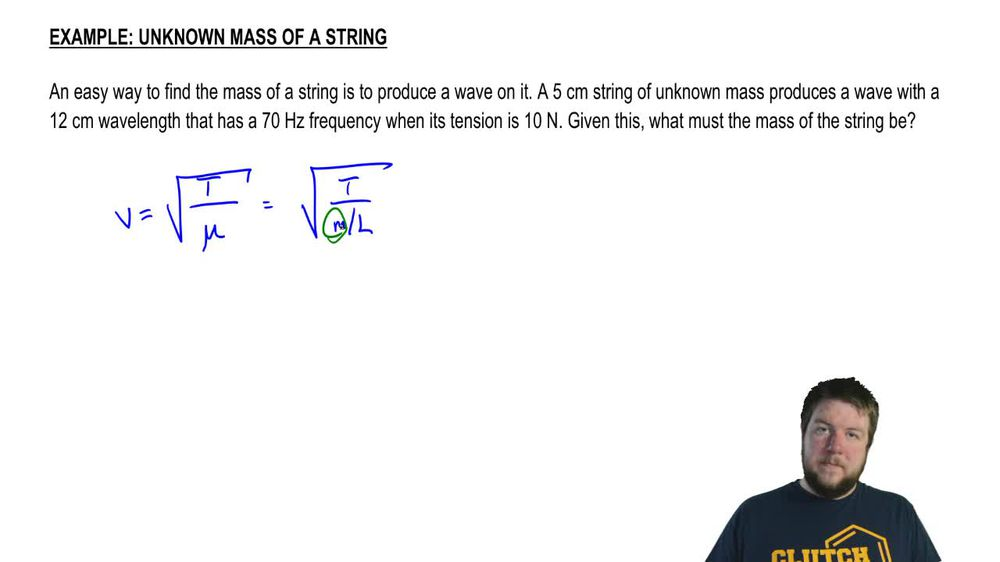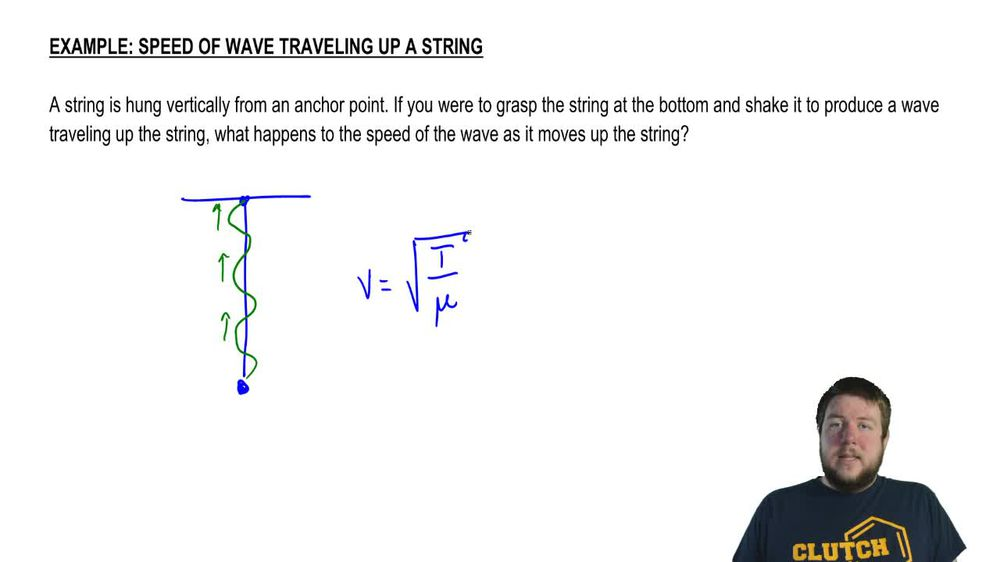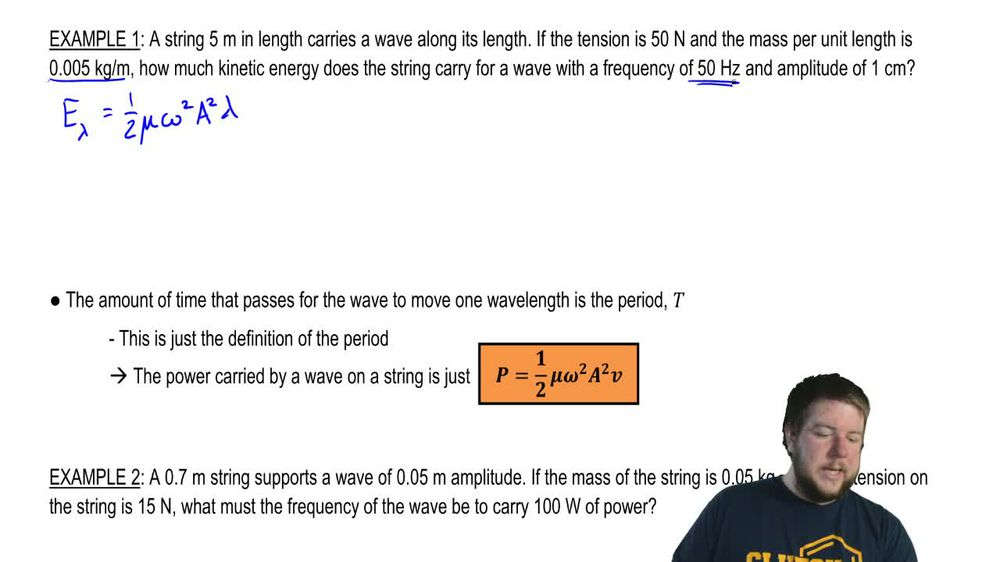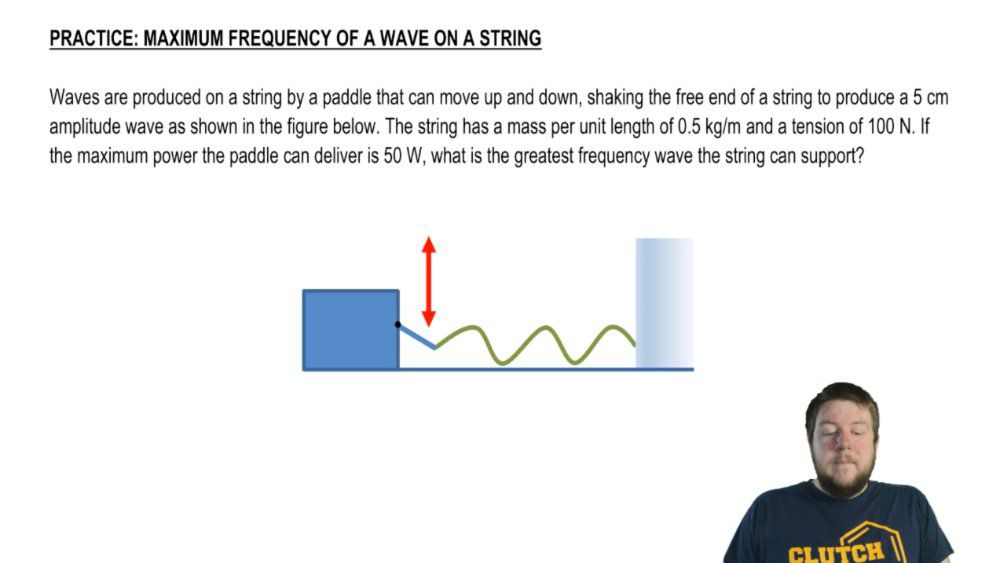Start typing, then use the up and down arrows to select an option from the list.
1. 18. Waves & Sound2. Velocity of Transverse Waves (Strings)# Energy Carried By Waves On A String

by Patrick Ford
142 views
1
20
1
Hey, guys. In this video, we want to talk about the energy carried by waves on a string. All right, let's get to it. Waves on a string carry both kinetic and potential energy. They obviously carry kinetic because as a wave which has sorry as a wave on a string that string has masked as it propagates it has a velocity and has mass. So it has kinetic energy. But when the string is just flat static, no waves on it when you whip it, that whip stretches the string a little bit to produce a wave. That wave has some stretching this in that in that string. So it also carries some potential energy with it as well. Okay, The kinetic energy is due to the motion off the string. Sorry. Wrong color. Kinetic energy is due to the motion of the string and the potential energy due. The potential energy is due to stretching of the stream. Okay, This energy depends upon three things. It depends upon the frequency of the wave. It depends upon the amplitude of the wave and it depends upon the wavelength of the waves. So pretty much the three main characteristics of a wave. All right. Now it turns out that the kinetic energy and the potential energy are the same for a wave on a string. Okay, They are both given by the same equation given right here in our orange box, where the variables mu, Omega A and Lambda are the same as they have been so far. The total energy per unit wavelength is just gonna be the some of the kinetic energy and the potential energy per unit wavelength. What I mean by per unit wavelength is this is the energy carried by a single wavelength. Okay, the energy per unit wavelength is just double this, right? It's this plus itself. So it's one half mu Omega squared, a squared lambda. All right, let's do a quick example. A string 5 m in length carries a wave along its length. If the tension is 50 Newtons and the mass per unit length is kg per meter. How much kinetic energy does the string carry for away with the frequency of 50 hertz and an amplitude of one centimeter? So we want to find first the energy per wavelength. Then we'll find the total energy this is one half mu Omega squared a squared lambda. So we know Mu Mu was given to us the mass per unit length. Okay, the frequency is given to us so we can find the angular frequency pretty easily. The amplitude is given to us. The only thing that's not given to us is the wavelength. The wavelength. We do have to calculate using our wave equation. This means that the wavelength is f over V. The question is, what's the speed of the wave? Well, since this is a wave on a string with speed is just the tension divided by the mass premium length square rooted. The tension, as we're told, is 50 Newtons. The mass print length is 500. and this whole thing equals 10. Sorry, 100 meters per second. The answer in the square root is 10,000 square of 10. 0 is 100. So this means that the wavelength is hertz, right? The frequency divided by 100 m per second, which is Oh, sorry. This is backwards. I messed up on this equation right here. This equation is V divided by f not F divided by V. So This is 100 divided by 50 which is 2 m. Okay, now we have everything we need to know to solve. For the energy per unit wavelength, that's one half 0.5 was the mass per unit length. The angular frequency is just two pi times 50 hertz, right? Was the frequency squared times the amplitude 01 m squared. Everything needs to be an s I units times the wavelength which was m. And this is 0.49 jewels. Okay, but this is not what the question asked. The question asked how much energy is carried by the string. Now this is the energy for a single wavelength. So the question is, how many wavelengths does the string have? Well, the string is 5 m in length and if the wavelength is 2 m thin, the whole string carries 2.5 wavelengths. So the total energy he's going to be too 0.5 Sorry. 2.5 times the energy per unit wavelength, which is 1.22 jewels. Okay, now something else you could ask is how much power is carried by the string. Well, we know the energy per unit wavelength. And how long does it take for a wavelength to pass just the period. So if I do thought, if I divide the energy period of wavelength by the period, I get the power, okay. And what we have is we have this last Lambda divided by the period, which gives us the velocity. And this is the power period. This isn't the power per unit wavelength. This is the total power. If you, for instance, asked for two times the energy or the energy per two wavelengths, you'd have to divide it by two periods. So that tude canceled from the numerator and the denominator. This power is not dependent on number of wavelength. This is just the power. All right, So a 0.7 m string supports a wave of 0.5 m amplitude. If the mass of the string is 0.5 kg and the tension in the string is Newton's, what must the frequency of the wave be to carry 100 watts of power? Since we're dealing with power, we'll start there. Windows is one half mu Omega squared, eight squared times the okay. We know the tension in the string. We know the mass per unit. We know the mass of the string, and we know the length of the string, so we can easily find the speed right. The speed is just the square root of tea divided by mu, which is the square root of 15. Newton's divided by 05 kg, divided by seven. Right, That's the mass per unit length, and that is 14 meters per second. All right, now what we wanna know is the frequency, right? What must the frequency be? Toe have 100 watts of power. So we need to rearrange our power equation to solve for frequency. The frequency in this equation is actually the angular frequency, but we can always convert that toe linear frequency later. So this becomes omega squared is to pee. I multiply the two up the two from the denominator up, and everything else goes into the denominator mu. Yeah, a squared Z. Okay, so omega is the square root of two p over mu, a squared V, which is gonna be two times the powers 100 watts. The mass per unit length, as I said was the mass 5 kg divided by the length. 7 m, the amplitude. We're told this 70.5 m and the speed we found as 14.5. Okay, so this whole thing becomes in verse seconds. The last thing we need to do is we need to take this number, which is angular frequency and convert it into linear frequency. Don't forget that linear frequency is angular frequency divided by two pi. So this is 879 divided by two pi, which is about 280 hertz. All right, that wraps up our discussion into the energy carried by waves on a string. Thanks for watching guys.